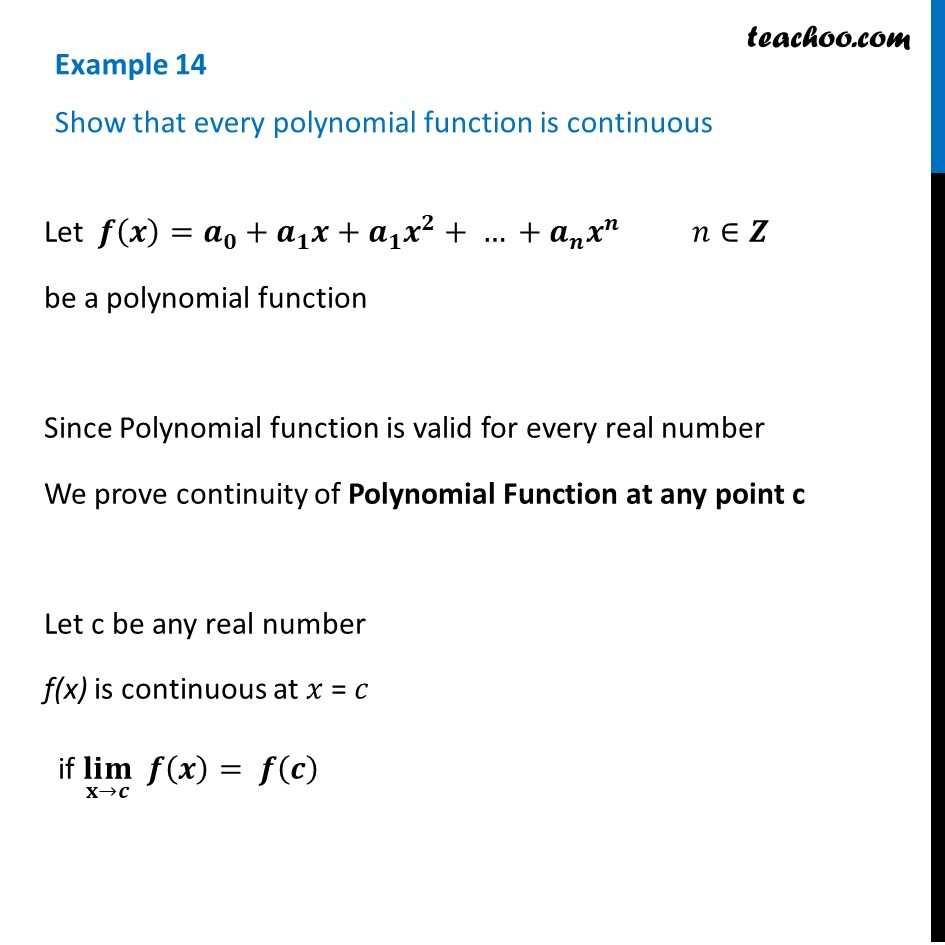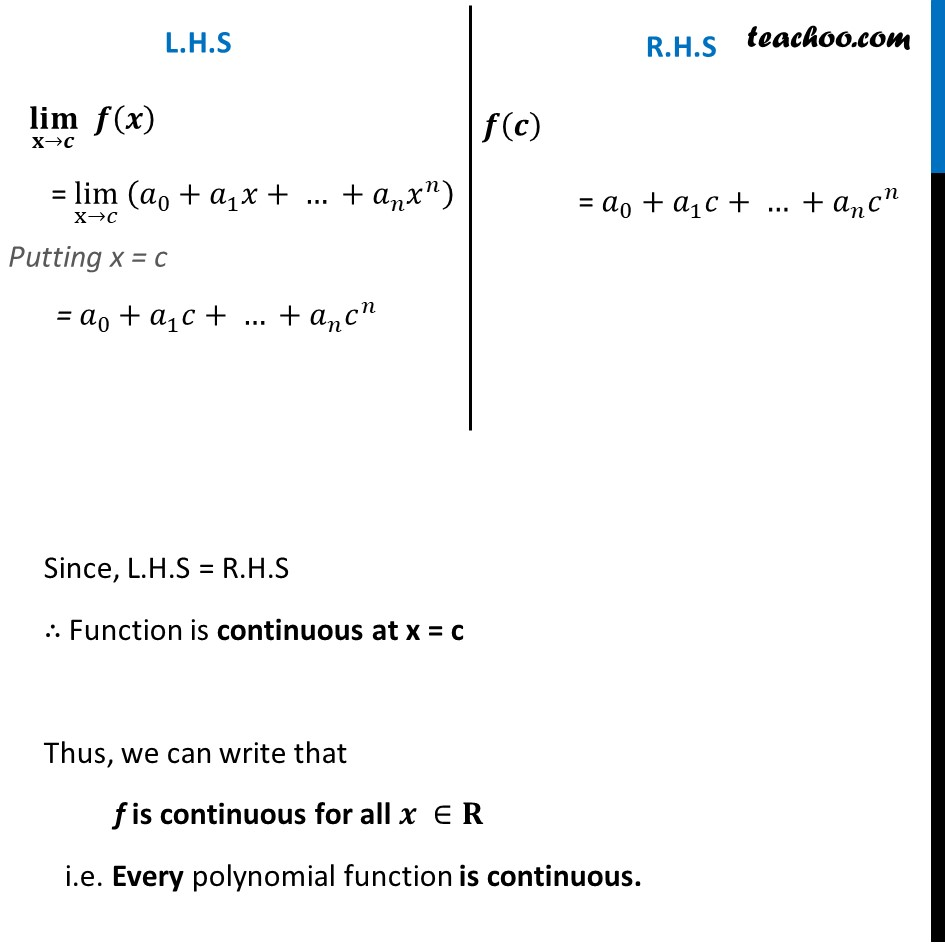Examples

Chapter 5 Class 12 Continuity and Differentiability
Serial order wiseLearn in your speed, with individual attention - Teachoo Maths 1-on-1 Class

### Transcript

Example 14 Show that every polynomial function is continuousLet 𝒇(𝒙)=𝒂_𝟎+𝒂_𝟏 𝒙+𝒂_𝟏 𝒙^𝟐+ … +𝒂_𝒏 𝒙^𝒏 𝑛∈𝒁 be a polynomial function Since Polynomial function is valid for every real number We prove continuity of Polynomial Function at any point c Let c be any real number f(x) is continuous at 𝑥 = 𝑐 if (𝐥𝐢𝐦)┬(𝐱→𝒄) 𝒇(𝒙)= 𝒇(𝒄) L.H.S (𝐥𝐢𝐦)┬(𝐱→𝒄) 𝒇(𝒙) = lim┬(x→𝑐) " " (𝑎_0+𝑎_1 𝑥+ … +𝑎_𝑛 𝑥^𝑛 ) Putting x = c = 𝑎_0+𝑎_1 𝑐+ … +𝑎_𝑛 𝑐^𝑛 R.H.S 𝒇(𝒄) = 𝑎_0+𝑎_1 𝑐+ … +𝑎_𝑛 𝑐^𝑛 Since, L.H.S = R.H.S ∴ Function is continuous at x = c Thus, we can write that f is continuous for all 𝒙 ∈𝐑 i.e. Every polynomial function is continuous.